MU 207 Midterm Study Guide

Office hours 4:30 - 5:30 pm Tues

MacLaurin A177

Source materials:  CLASS NOTES, TEXT, VIDEOS

Introduction to acoustics. Sinusoids, Hooke's law, SHM

Vibrating spring vs. vibrating string, velocity v of a wave on a string:(T = tension in Newtons, µ = mass per unit length)

Amplitude, frequency, period, phase

wavelength:   v = f  λ

T = 1/f or f = 1/T (T is the period)

Harmonics, resonance, standing waves, difference between modes and nodes

Fourier Theory, Time domain vs. frequency domain

Digital Audio, Sampling, Information theory: Nyquist/Shannon

Sampling rate, aliasing

Quantization, dynamic range ("6 dB per bit"), mp3, perceptual coding vs lossless compression

How does a computer make music?

The physics and mathematics of intervals, cents, tuning systems:  just, Pythagorean, meantone, equal tempered
pythagorean and syntonic commas

Psychoacoustics : anatomy of auditory system, tonotopicity, jnd, critical bands, missing fundamental, Seebeck's siren (1841, confirmed by Schouten in 1940)
Fletcher-Munson curves

ANIMATION: Auditory Transduction

The Science of Musical Scound (coursepack):

Chapter 3 is about sine waves, harmonics, harmonic series (p 44), resonant modes (standing waves) and Fourier Theory.

Chapter 4 is about tuning systems, and has a slightly different take than the way I presented it, but this chapter describes just intonation

Just intonation is specifically derived in Figure 4-5 on page 70, cents on page 72.

Chapter 5, pages 78-82 are about critical bands

Chapter 6, page 92-95 is about virtual pitch (Jan Schouten)

Chapter 7 is about the anatomy of the auditory system, p 112 discusses critical bands again briefly.
Chapter 8, page 123 describes Fletcher-Munson equal-loudness curves

Not on midterm:

MIDI and computer music systems.  (Appendix G)

Musique Concrete, Early Electronic Music

Reference (not necessarily all used or referred to)• At room temperature (68° Fahrenheit), the velocity of sound in air is: 343 meters/second = 769 mph = 1,128 feet/second  = 769 miles/hour.
In water  the speed of sound is: 1,497 meters/second.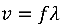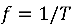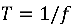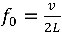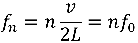where v is velocity, L is length of the string or air column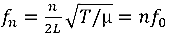where v = velocity of a disturbance passing along a string, T is tension of the string, µ is mass per unit length (thickness)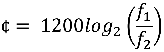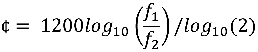(if your calculator can't do log base 2)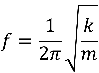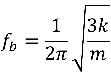"6 dB per bit"

1¢ is defined as 1/100th of an equal-tempered semitone

Quarter-comma Meantone temperament:
C         D-½δ              E-δ                  F+¼δ              G-¼δ              A-¾δ               B-δ                C